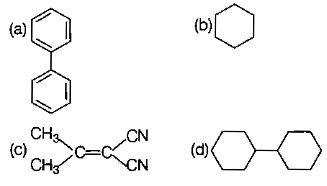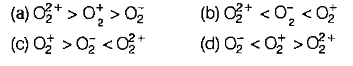The hybridisations of atomic orbitals of nitrogen in  and ${\mathrm{NH}}_{4}^{+}$ are

(a) sp, sp3 and sp2               (b) sp2, sp3 and sp

(c) sp, sp2 and sp3               (d) sp2, sp and sp3

Concept Questions :-

Hydrogen Bonding
High Yielding Test Series + Question Bank - NEET 2020

Difficulty Level:

Which of the following pairs of ions is isoelectronic and isostructural?

1. a,d

2. b,d

3. a,c

4. b,d,c

Concept Questions :-

Hybridisation
High Yielding Test Series + Question Bank - NEET 2020

Difficulty Level:

The correct geometry and hybridisation for XeF4 are

(a) octahedral, sp3d2

(b) trigonal bipyramidal, sp3d2

(c) planar triangle, sp3d3

(d) square planar, sp3d2

Concept Questions :-

Hybridisation
High Yielding Test Series + Question Bank - NEET 2020

Difficulty Level:

In which of the following molecules, all atoms are coplanar ?Concept Questions :-

Hybridisation
High Yielding Test Series + Question Bank - NEET 2020

Difficulty Level:

Predict the correct order among the following.

(a) lone pair-lone pair>bond pair-bond pair>lone pair-bond pair

(b) bond pair-bond pair>lone pair-bond pair>lone pair-lone pair

(c) lone pair-bond pair>bond pair-bond pair>lone pair-lone pair

(d) lone pair-lone pair>lone pair-bond pair>bond pair-bond pair

Concept Questions :-

V.S.E.P.R
High Yielding Test Series + Question Bank - NEET 2020

Difficulty Level:

The pair of electron in the given carbanion, CH3C$\equiv$C-, is present in which orbitals?
(a) sp3
(b) sp2
(c) sp
(d)2p

Concept Questions :-

Hybridisation
High Yielding Test Series + Question Bank - NEET 2020

Difficulty Level:

Consider the molecules CH4, NH3 and H2O. Which of the given statements is false?
(a) The H-O-H bond angle in H2O is larger than the H-C-H bond angle in CH4
(b) The H-O-H bond angle in H2O is smaller than the H-N-H bond angle in NH3
(c) The H-C-H bond angie in CH4 is larger than the H-N-H bond angle in NH3
(d) The H-C-Hbond angle in CH, the H-N-H bond angle in NH3 and the H-O-H bond angle in H2O are all greater than 90°

Concept Questions :-

V.S.E.P.R
High Yielding Test Series + Question Bank - NEET 2020

Difficulty Level:

Which of the following species contains equal number of $\mathrm{\sigma }$ and $\mathrm{\pi }$ bonds?

(a) ${\mathrm{HCO}}_{3}^{-}$                                    (b) XeO4

(c) (CN)2                                      (d) CH2(CN)2

Concept Questions :-

Hybridisation
High Yielding Test Series + Question Bank - NEET 2020

Difficulty Level:

Which of the following pairs of ions are isoelectronic and isostructural?

Concept Questions :-

Types of Bonding
High Yielding Test Series + Question Bank - NEET 2020

Difficulty Level:

The correct bond order in the following species isConcept Questions :-

M.O.T